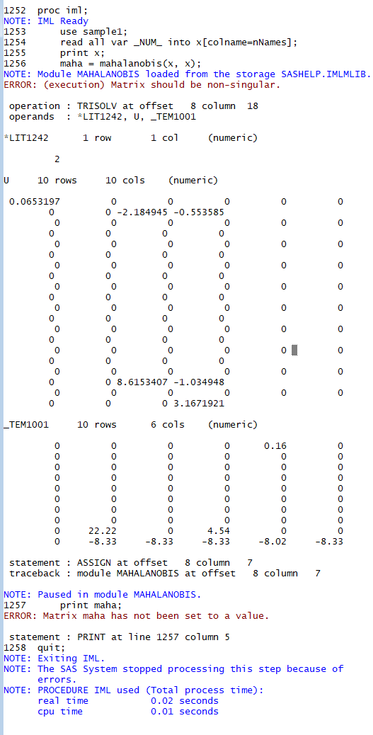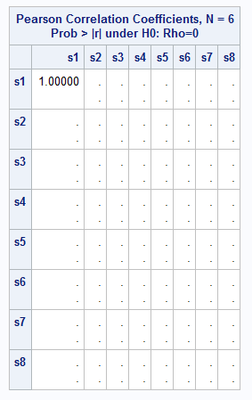## How do I compute MAHALANOBIS DISTANCE with Proc IML when Matrix is Singular?

I do Cosine Similarity with PROC DISTANCE, and it always returns a solution (with missing values when it does not apply).

As Mahalanobis distance is not an option in PROC DISTANCE, I'm using Proc IML following your posts. But I get the error of "Matrix should be non-singular". I don't get this error when using PROC DISTANCE for cosine similarity using the same dataset.

I have to compute the Cosine Similarity and Mahalanobis Distance for super large datasets, and I don't know how to avoid that error, or to force it.

Example of what I'm trying:

data sample;
infile datalines;
input gvkey:8. s1:8. s2:8. s3:8. s4:8. s5:8. s6:8. s7:8. s8:8. s9:8. s10:8.;
datalines;
1000 0 0 0 0 0 0 0 0 0 8.33
1004 0 0 0 0 0 0 0 0 22.22 0
1010 0 0 0 0 0 0 0 0 0 0
1012 0 0 0 0 0 0 0 0 4.54 0
1013 0.16 0 0 0 0 0 0 0 0 0.31
1016 0 0 0 0 0 0 0 0 0 0
;;;;
run;

* Cosine similarity (convert gvkey to string for proc distance);
data sample1(drop=gvkey rename=(firm=gvkey));
retain firm;
set sample;
firm = put(gvkey,best8.);
run;

* Proc distance for Cosine Similarity;
proc distance data=sample1 out=Cos method=COSINE shape=square replace;
var ratio(s1--s10);
id gvkey;
run;
proc print data=cos (obs=10); run;

proc iml;
use sample1;
read all var _NUM_ into x[colname=nNames];
print x;
maha = mahalanobis(x, x);
print maha;
quit;

Output for print data=cos (using proc distance)Error from Proc IML:5 REPLIES 5

## Re: How do I compute MAHALANOBIS DISTANCE with Proc IML when Matrix is Singular?

To get a Mahalanobis distance, you must first invert the matrix. However, your matrix has 10 columns and only 6 records, this matrix cannot be inverted. You need more records than columns — and other conditions also exist to determine if a matrix is invertible — for example you have several columns that are identically all zero, this also prevents the matrix from being inverted.

So, if you delete the columns that are all zero, you can get Mahalanobis distances. You could also use the output from PROC PRINCOMP and compute the Mahalanobis distance from the scores of the dimensions that PRINCOMP shows have non-zero eigenvalues.

--
Paige Miller

## Re: How do I compute MAHALANOBIS DISTANCE with Proc IML when Matrix is Singular?

Thanks Paige. The dataset follows the structure needed for Mahalanobis distance:

x specifies an $n\times p$ numerical matrix that contains $n$ points in $p$-dimensional space.

As can be seen in the description of the function: https://support.sas.com/documentation/cdl/en/imlug/65547/HTML/default/viewer.htm#imlug_modlib_sect01...

## Re: How do I compute MAHALANOBIS DISTANCE with Proc IML when Matrix is Singular?

The dataset follows the structure needed for Mahalanobis distance:

I really don't know what you mean by this.

The data set you provided can not be inverted, for the reasons I mentioned, so no Mahalanobis distance can be computed. N has to be greater than or equal to P. Linear combinations of columns cannot be exactly equal to linear combinations of other columns.

So what do you mean?

--
Paige MillerKsharp
Super User

## Re: How do I compute MAHALANOBIS DISTANCE with Proc IML when Matrix is Singular?

You cannot compute the Mahalanobis distance for your sample data because the correlation/covariance matrix of s1-s8 is fully degenerate. In this case, you cannot invert the correlation or covariance matrix to compute the distances. Computing and utilizing a generalized inverse is not even possible in this case.

If you run PROC CORR, you will see those correlations cannot be computed due to all the values of s1-s8 being 0.

ods output PearsonCorr=sample_corr;
proc corr data=sample pearson;
var s1-s8;
run;From The DO Loop